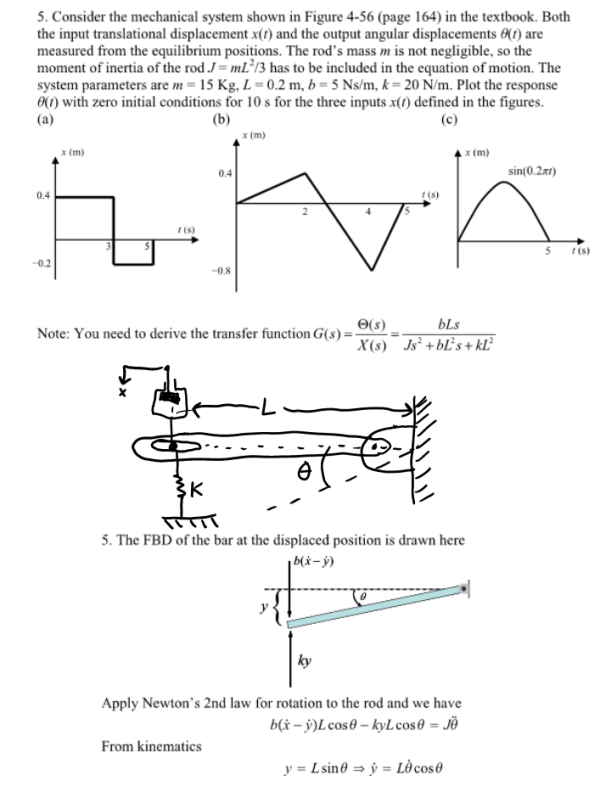Consider the mechanical system shown in the figure in the textbook. Both the input translational displacement x(t) and the output angular displacements theta(t) are measured from the equilibrium positions. The rod's mass m is not negligible, so the moment of inertia of the rod J=mL^2/3 has to be included in the equation of motion. The system parameters are m=15 kg, L=0.2 m, b=5 Ns/m, k=20 N/m. Plot the response theta(t) with zero initial conditions for 10s for the three inputs x(t) defined in the figures.Consider the mechanical system shown in the figure in the textbook. Both the input translational displacement x(t) and the output angular displacements theta(t) are measured from the equilibrium positions. The rod's mass m is not negligible, so the moment of inertia of the rod J=mL^2/3 has to be included in the equation of motion. The system parameters are m=15 kg, L=0.2 m, b=5 Ns/m, k=20 N/m. Plot the response theta(t) with zero initial conditions for 10s for the three inputs x(t) defined in the figures.

System Dynamics Page 2 dynamics dynamics dynamics dynamics dynamics dynamics dynamics System dynamics Page 3